# Battery Storage System Design Using PWM Current and Voltage Controllers

Battery Storage System Design Using PWM Current and Voltage Controllers
Department of Electrical Engineering, University Badji Mokhtar, Annaba 23000, Algeria

Corresponding Author Email:
sghoudelbourk@yahoo.fr
Page:
195-200
|
DOI:
https://doi.org/10.18280/ejee.240404
19 June 2022
|
Accepted:
1 August 2022
|
Published:
31 August 2022
| Citation

OPEN ACCESS

Abstract:

Today, energy storage systems are strongly present both in industry and for individual applications, especially in on-board systems where an autonomous source of energy is required for power. Energy needs as well as the search for efficient means for its storage have become a very important research axis. Solar battery storage allows to store electricity generated from the sun via solar panels to ensure power supply in all circumstances (day or night, clear or overcast sky). This work focuses on modeling and dynamic simulation of a photovoltaic system with a Lithium Ion battery storage system (LI-BSS). Battery charge and discharge is performed using PWM current and voltage controllers using DC/DC bidirectional management converter BMC.

Keywords:

photovoltaic system, lithium-ion battery, boost chopper, bidirectional DC-DC converter, PWM controller

1. Introduction

Due to population growth, urbanization and development global energy demand continues to rise. Non-renewable energy defines resources that are depleted faster than they are replenished. However renewable resources are permanently available to us. Renewable resources have the disadvantage of being sparsely concentrated, and their intermittency poses some problems for isolated applications .

Solar energy is particularly suitable for generating electricity in isolated sites, or in micro-grids. It is then often associated with batteries, which make it possible to ensure the storage of energy in case of excess production, or to compensate for the temporary lack of power during peak consumption .

A storage element is then required to supply the customer. There are several methods of storing energy. The most important are batteries, super capacitors and fuel cells. Adding a solar battery system is a great way to use renewable energy to increase independence from the grid [3, 4].

This work focuses on modeling, dynamic simulation and integration of a lithium ion battery storage system (LI-BSS). Modelling and simulation of the basic characteristics of photovoltaic (PV) modules controlled by adaptive P&O maximum power point tracking (MPPT) method and connected to a DC-DC boost converter is done in the first section. An energy storage module (battery) connected to a bidirectional DC-DC with charge and discharge PWM controller has been studied, implemented and discussed in the last section.

2. PV Module Mathematical Model

As presented in Figure 1, the PV module is basically modeled by using a current source, a diode and two series and parallel resistors .

## 1.png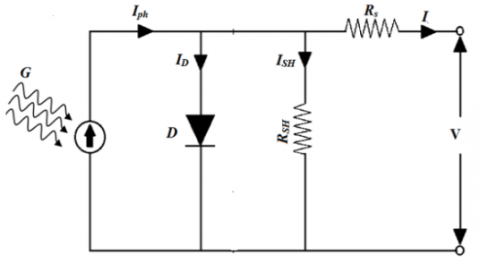Figure 1. Equivalent circuit of PV module

Photonic generated current basically depends on irradiance and temperature.

$I_{p h}=I_{s c}+k_i\left(T-T_{r e f}\right)\left(\frac{G}{1000}\right)$          (1)

The short-circuit current Isc is defined by:

$I_{s c}=I_s=I_{p h}$

The module-reverse saturation current Irs and I0 which varies with cell temperature are given by:

$I_{r s}=I_{s c} /\left[\exp \left(\frac{q V_{o c}}{N_s k n T}\right)-1\right]$              (2)

$I_o=I_{r s}\left[\frac{T}{T_r}\right]^3 \exp \left[\frac{q E_g}{n k}\left(\frac{1}{T}-\frac{1}{T_r}\right)\right]$                (3)

The current voltage characteristics will be:

$I=I_{p h}-I_s\left(e^{\frac{q\left(V+IR_s\right)}{n K T_c}}-1\right)-\frac{V+I R_s}{R_{s h}}$                (4)

The PV cell output voltage and power depend on the generated current value which again depends on the solar irradiance and temperature. Figure 2 shows P-V and I-V characteristics for irradiation and temperature variations. The PV array type simulated in Matlab/Simulink software is: 1Soltech 1STH-215-P with 2 series modules and 2 parallel strings. Each module is composed by 96 series cells. The various parameters of PV module are listed in Table 1.

Table 1. Parameters of the solar module

 Open circuit voltage (Voc) 36.3 V Short-circuit current (Isc) 7.84 A Voltage at MPP (Vmp) 29 V Current at MPP (Imp) 7.35 Maximum Power (Pm) 213.15 w Ns 96

This panel in standard conditions STC (G =1KW/m2, T= 25℃) can provide a power Pmp of 852 (W) which corresponds to a terminal voltage Vpvm of 58 (V). It is clear that current and power increase with increasing solar irradiation. Voltage and power decrease in the case of temperature rise.

## 2.pngFigure 2. a. P-V and I-V characteristics for irradiation variation

## 2b.png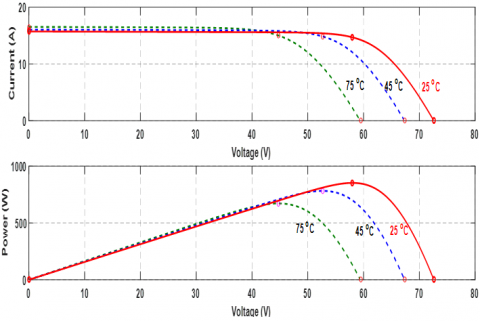Figure 2. b. P-V and I-V characteristics for temperature variation

An MPPT control associated with an adaptation static converter, makes a GPV to operate and to permanently produce the maximum of its power. Thus, whatever the meteorological conditions (temperature and irradiation). The control of the converter places the system at the maximum operating point (Vmp and Imp). The variable perturbation step size which depends on power changes can reduce the most drawbacks of the conventional P&O method and track quickly the MPP under fast varying atmospheric conditions [6, 7]. Flowchart of the adaptive P&O MPPT algorithm is presented on Figure 3.

## 3.pngFigure 3. Flowchart of the adaptive P&O MPPT algorithm

First various simulations are carried out for a PV system implemented in Matlab/Simulink software which is built mainly of a PV panel, a resistive load and a Boost chopper controlled by the proposed adaptive P&O MPPT algorithm. Figure 4 shows PV and load power with and without MPPT. Figure 5 illustrates power and voltage of the PV panel for fast irradiation variation. Simulation results clearly show the effectiveness of the applied control.

## 4a.png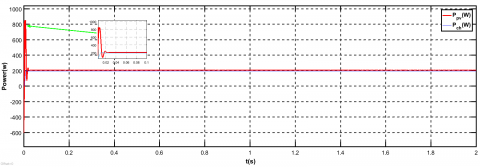-a-

## 4b.png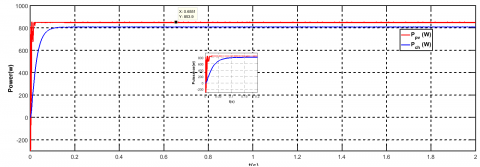-b-

Figure 4. PV and load power. a- without MPPT, b- with adaptive P&O MPPT

## 5a.png## 5b.png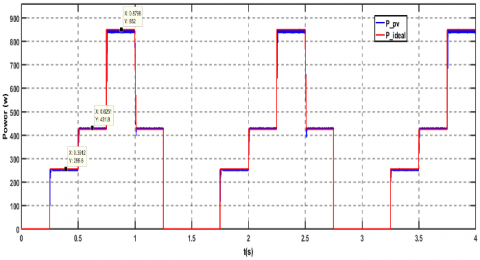Figure 5. Power and voltage of PV panel for irradiation variation

Table 2 shows comparison of MPPT technique used in this paper with conventional ones to track the MPP [6, 7].

Table 2. Comparaison of different MPPT techniques

 Performance criteria P&O INC Adaptive P&O Number of sensing parameters 1 Vpv 2 Vpv,Ipv 2 Vpv,Ipv complexity less more less Accuracy to track MPP Less time but high oscillations more time but low oscillations Fast with little oscillations
4. Dynamic Simulation and Integration of a Lithium Ion Battery Storage System (LI-BSS)

The battery-operated photovoltaic system designed in this work includes a solar panel that ensures the power supply of the load and the charging of the battery in the period of sunlight. An accumulator battery which ensures the storage (daily/seasonal) of electrical energy and the supply of the load in periods of darkness or when the energy required by the load is greater than that which can be supplied by the panel (Figure 7).

A DC/DC bidirectional management converter BMC which is designed and implemented for the Lithium Ion battery storage system (LI-BSS) using PWM current and voltage controllers. Solar regulator used in photovoltaic systems is used to protect the battery against overcharge and deep discharge phenomena to guarantee the storage device’s safety, reliability, and long life [8, 9].

5. Typical Discharge Pattern of the LI-BSS

The considered model LI-BSS mathematical model can be generalized by two main equations:

Discharge mode (i* > 0):

$f_1(i t, i *, i)=E_0-K \cdot \frac{Q}{Q-i t} \cdot i *-K \cdot \frac{Q}{Q-i t} \cdot i t+A\cdot \exp (-B \cdot i t)$          (5)

Charge mode (i* < 0):

$f_2(i t, i *, i)=E_0-K \cdot \frac{Q}{i t+0.1 \cdot Q} \cdot i *-K \cdot \frac{Q}{Q-i t}\cdot i t+A \exp (-B \cdot i t)$           (6)

The Battery block used is taken from Simulink library. It is a generic dynamic model parameterized to represent most popular types of rechargeable batteries. The equivalent circuit is shown in Figure 6.

## 6.png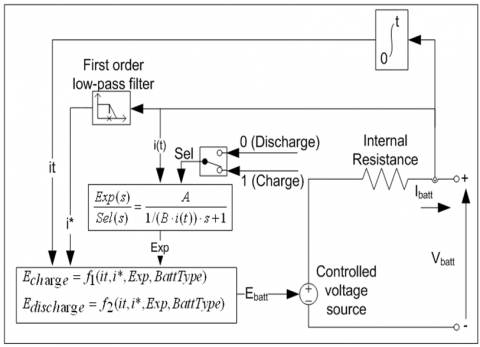Figure 6. Dynamic model of the battery

## 7.png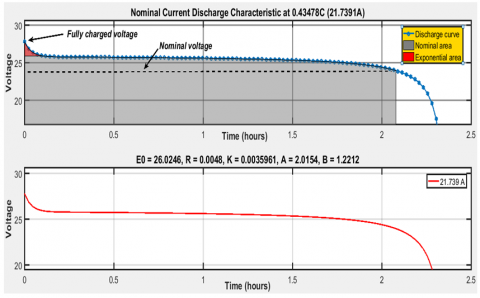Figure 7. Nominal current discharge characteristic

The purpose of the simulations is to charge and then discharge the battery at constant current considering the accumulator data given in Table 3.

Table 3. Parameters of a lithium ion battery

## t3.png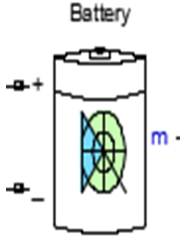Nominal Voltage (V)

24

Rated Capacity (Ah)

50

Initial State-Of-Charge (%)

45

Fully charged Voltage (V)

27.93

Nominal discharge current (A)

21.7

A typical discharge curve is composed of three sections, as shown in Figure 7 The first section represents the exponential voltage drop when the battery is charged. Depending on the battery type, this area is more or less wide. The second section represents the charge that can be extracted from the battery until the voltage drops below the battery nominal voltage. Finally, the third section represents the total discharge of the battery, when the voltage drops rapidly.

Simulink model of Photovoltaic system with the Lithium Ion Battery storage using Bidirectional DC-DC converter is shown in Figure 8.

## 8.png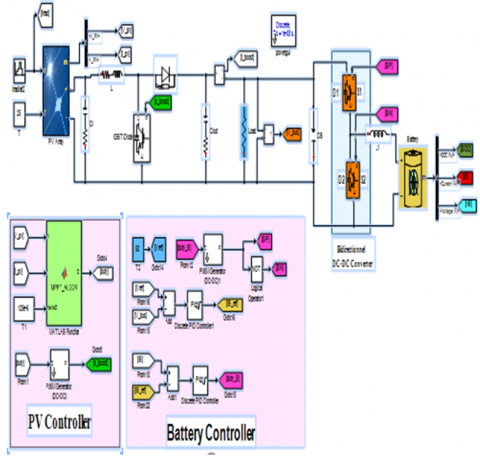Figure 8. Simulink model of photovoltaic system with the lithium ion battery storage using bidirectional DC-DC converter

## 9.pngFigure 9. PWM controllers design for bidirectional DC-DC converter

Two PI controllers are used to manage battery charging and discharging (Figure 9). The first provides reference current from voltage load control. The second controls discharge current of the battery and generates pulses for switches of the bidirectional DC-DC converter for Buck operation (charge mode) and for Boost operation (discharge mode) [10, 11].

Figure 10 shows evolution of state of charge SOC, voltage, current of the Lithium Ion battery and power generated by PV panel for irradiation variation. It can be seen that when the irradiation of the PV array is able to generate enough voltage, the PV array will charge the battery through the bidirectional DC-DC converter and also supply power to the load during this time.

## 10a.png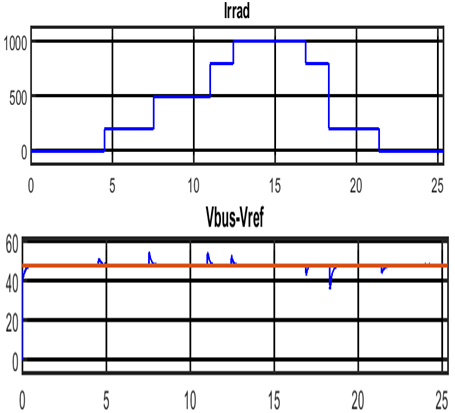## 10b.png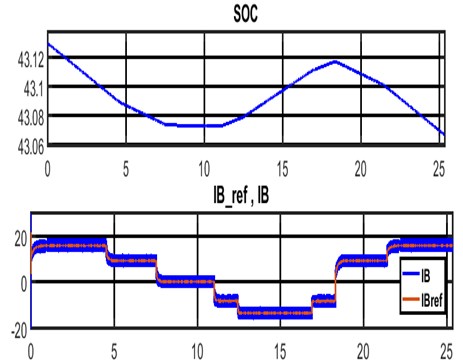## 10.png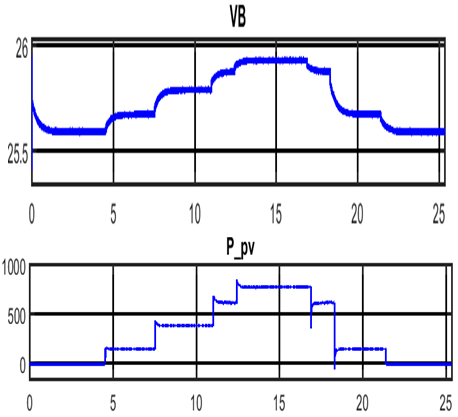Figure 10. Evolution of battery voltage, current, state of charge SOC and PV output power during Irradiation variation

## 11a.png## 11b.png## 11c.pngFigure 11. Evolution of battery voltage, current, state of charge SOC and PV output power during Irradiation variation

For irradiation G=1000 w/m2 and G=800 w/m2 Ppv is greater than Pbattery, so PV panel supply the load and charge the battery. At night (G=0 w/m2) or for G=500 w/m2 and G=300 w/m2 Ppv is less than Pbattery, in this case the load is supplied by the battery. When the irradiation cannot generate sufficient voltage, the battery will power the load through the same bidirectional DC-DC converter, it will discharge through it. While the battery is charging, the battery voltage VB will increase. Because of this, charge current IB should decrease. When battery is in discharge mode the SOC is decreasing and the battery current is positive. Load voltage is around 48V.

Figure 11 shows dynamic performances of the solar storage system for temperature variation. It is clear that for this case there is one working mode it is the charge mode. The increase in temperature does not alter the working of the system too much, contrary the variation in irradiation.

6. Conclusion

The work presented in this article deals with the study of a photovoltaic system energetically coupled to storage batteries ensuring continuous energy availability. This system operates at its optimum power with the use of an adaptive P&O MPPT algorithm applied to the step up Boost converter. The maximum power point is being successfully tracked. Simulation and analysis of closed loop control of bidirectional DC-DC buck-boost converter using two PWM current and voltage regulators was performed in both charge and discharge modes.

Nomenclature
 G solar irradiance T cell p-n junction temperature Rsh equivalent shunt resistance Rs equivalent series resistance I output current V output voltage Iph photo generated electric current Isc short circuit current Id current at diode D K Boltzman’s constant Q electron charge Is diode reverse bias saturation current n diode ideality factor Ns Number of series cells Eg Band gap energy of the semi conductor E0 Constant battery voltage (V) i Battery current (A) i* Low frequency current dynamics (A) it Extracted capacity (Ah) Q Maximum battery capacity (Ah) A Exponential voltage (V) B Exponential capacity (Ah)−1 K Polarization resistance (Ohms)
References

 Zhang, G.Q., Dai, Y.J., Cui, J.M. (2016). Design and realization of a bi-directional DC-DC converter in photovoltaic power system. International Forum on Energy, Environment and Sustainable Development. https://doi.org/10.2991/ifeesd-16.2016.197

 Tummuru, N.R., Mishra, M.K., Srinivas, S. (2015). Dynamic energy management of hybrid energy storage system with high-gain PV converter. IEEE Transactions on Energy Conversion, 30(1): 150-160. https://doi.org/10.1109/TEC.2014.2357076

 Restrepo, M.J., Kazerani, M., Cañizares, C.A. (2018). Modeling and testing of a bidirectional smart charger for distribution system EV integration. IEEE Transactions on Smart Grid, 9(1): 152-162. https://doi.org/10.1109/TSG.2016.2547178

 Tomar, P.S., Sharma, A.K., Hada, K. (2017). Energy storage in DC microgrid system using non-isolated bidirectional soft-switching DC/DC converter. 6th International Conference on Computer Applications in Electrical Engineering-Recent Advances. https://doi.org/10.1109/CERA.2017.8343370

 Bahari, M.I., Tarassodi, P., Naeini, Y.M. (2016). Modeling and simulation of hill climbing MPPT algorithm for photovoltaic application. Symposiom Speedam. https://doi.org/10.1109/SPEEDAM.2016.7525990

 Lian, K.L., Jhang, J.H., Tian, I.S. (2014). A maximum power point tracking method based on perturb-and-observe combined with particle swarm optimization. IEEE Journal of Photovoltaics, 4(2): 626-633. https://doi.org/10.1109/JPHOTOV.2013.2297513

 Manisha, K., Kumar, N. (2021). Comparative study of different MPPT techniques for PV system. International Conference for Emerging Technology. https://doi.org/10.1109/INCET51464.2021.9456271

 Kollimalla, S.K., Mishra, M.K. (2013). Novel adaptive P&O MPPT algorithm for photovoltaic system considering sudden changes in weather condition. Proc. Int. Conf. Clean Elect. Power, pp. 653-658. https://doi.org/10.1109/TEC.2014.2320930

 Pahari, O.P., Subudhi, B. (2018). Integral sliding mode-improved adaptive MPPT control scheme for suppressing grid current harmonics for PV system. IET Renewable Power.Generation, 12(16): 1904-914. https://doi.org/10.1049/IET-RPG.2018.5215

 Tytelmaier, K., Husev, O., Veligorskyi, O., Yershov, R. (2016). A review of non-isolated bidirectional DC-DC converters for energy storage systems. International Young Scientists Forum on Applied Physics and Engineering, pp. 22-28. https://doi.org/10.1109/YSF.2016.7753752

 Mirzaei, A., Forooghi, M., Ghadimi, A., Abolmasoumi, A.H., Riahi, M.R. (2017). Design and construction of a charge controller for standalone PV/battery hybrid system by using a new control strategy and power management. Solar Energy, 149: 132-144. https://doi.org/10.1016/j.solener.2017.03.046